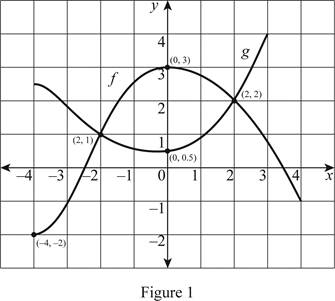# The value of f ( − 4 ) and g ( 3 ) .### Single Variable Calculus: Concepts...

4th Edition
James Stewart
Publisher: Cengage Learning
ISBN: 9781337687805### Single Variable Calculus: Concepts...

4th Edition
James Stewart
Publisher: Cengage Learning
ISBN: 9781337687805

#### Solutions

Chapter 1.1, Problem 2E

(a)

To determine

## The value of f(−4) and g(3) .

Expert Solution

The value of f(4)=2 and g(3)=4 .

### Explanation of Solution

Given:

Plot the points in the given graph as shown below in Figure 1.Calculation:

In Figure 1, x-axis represents the values of x and y-axis represents the values of f(x) and g(x) .

From Figure 1, it is noticeable that the point (4,2) is plotted on the graph f and the point (3,4) is plotted on the graph g.

Therefore, f(4)=2 and g(3)=4 .

(b)

To determine

### The values of x if f(x)=g(x) .

Expert Solution

At x=2 and 2 , the values of f(x) and g(x) are equal.

### Explanation of Solution

Observe that the curves f and g are intersecting at two points (2,1) and (2,2) . At these points, the values of f(x) and g(x) are equal.

Therefore, the value of f(x) and g(x) are equal at x=2 and x=2 .

(c)

To determine

### The value of x if f(x)=−1 .

Expert Solution

At f(x)=1 , the values of x are −3 and 4.

### Explanation of Solution

From Figure 1, it is obvious that the points (3,1) and (4,1) are located on the f graph.

If f(x)=1 , then the values of x are −3, 4.

That is, if y=1 , then the values of x are −3, 4.

Therefore, the values of x = −3, 4 when f(x)=1 .

(d)

To determine

### The decreasing interval of f.

Expert Solution

The decreasing interval of f is [0,4] .

### Explanation of Solution

From Figure 1, it is noticeable that the curve f is decreasing from 3 to −1 between x = 0 and x=4 .

Therefore, the decreasing interval of f is [0,4] .

(e)

To determine

### The domain and range of f.

Expert Solution

The domain of f is [4,4] and the range of f is [2,3] .

### Explanation of Solution

Since the domain of a function is the set of all x values of the graph, the domain is [4,4] and in interval notation, the domain is 4x4 .

Thus, the domain of the function f is [4,4] and in interval notation, it is 4x4 .

Since, the range of a function is the set of all y values of the graph, the range is [2,3] , and in interval notation, 2y3 .

Thus, the range of the function f is [2,3] and in interval notation, 4x4 .

(f)

To determine

### The domain and range of g.

Expert Solution

The domain of g is [4,3] and the range of g is [12,4] .

### Explanation of Solution

Since, the domain of a function is the set of all x values of the graph, the domain is [4,3] and in interval notation, 4x3 .

Thus, the domain of the function g is [4,3] and in interval notation 4x3 .

Since, the range of a function is the set of all y values of the graph, the range is [12,4] , and in interval notation, 12y4 .

Thus, the range of the function g is [12,4] and in interval notation, 12y4 .

### Have a homework question?

Subscribe to bartleby learn! Ask subject matter experts 30 homework questions each month. Plus, you’ll have access to millions of step-by-step textbook answers!